# Control of a Two-Tank System

This example shows how to use Robust Control Toolbox™ to design a robust controller (using D-K iteration) and to do robustness analysis on a process control problem. In our example, the plant is a simple two-tank system.

Additional experimental work relating to this system is described by Smith et al. in the following references:

• Smith, R.S., J. Doyle, M. Morari, and A. Skjellum, "A Case Study Using mu: Laboratory Process Control Problem," Proceedings of the 10th IFAC World Congress, vol. 8, pp. 403-415, 1987.

• Smith, R.S, and J. Doyle, "The Two Tank Experiment: A Benchmark Control Problem," in Proceedings American Control Conference, vol. 3, pp. 403-415, 1988.

• Smith, R.S., and J. C. Doyle, "Closed Loop Relay Estimation of Uncertainty Bounds for Robust Control Models," in Proceedings of the 12th IFAC World Congress, vol. 9, pp. 57-60, July 1993.

### Plant Description

The plant in our example consists of two water tanks in cascade as shown schematically in Figure 1. The upper tank (tank 1) is fed by hot and cold water via computer-controlled valves. The lower tank (tank 2) is fed by water from an exit at the bottom of tank 1. An overflow maintains a constant level in tank 2. A cold water bias stream also feeds tank 2 and enables the tanks to have different steady-state temperatures.

Our design objective is to control the temperatures of both tanks 1 and 2. The controller has access to the reference commands and the temperature measurements.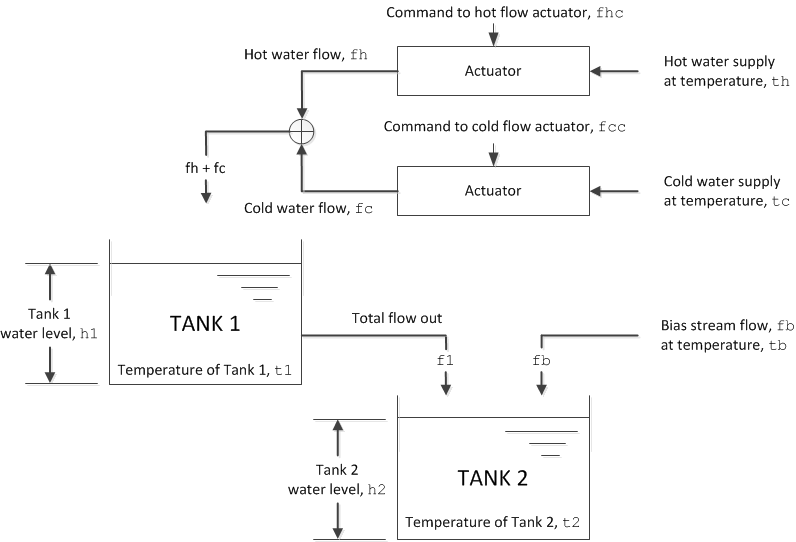Figure 1: Schematic diagram of a two-tank system

### Tank Variables

Let's give the plant variables the following designations:

• `fhc`: Command to hot flow actuator

• `fh`: Hot water flow into tank 1

• `fcc`: Command to cold flow actuator

• `fc`: Cold water flow into tank 1

• `f1`: Total flow out of tank 1

• `A1`: Cross-sectional area of tank 1

• `h1`: Tank 1 water level

• `t1`: Temperature of tank 1

• `t2`: Temperature of tank 2

• `A2`: Cross-sectional area of tank 2

• `h2`: Tank 2 water level

• `fb`: Flow rate of tank 2 bias stream

• `tb`: Temperature of tank 2 bias stream

• `th`: Hot water supply temperature

• `tc`: Cold water supply temperature

For convenience we define a system of normalized units as follows:

``` Variable Unit Name 0 means: 1 means: -------- --------- -------- -------- temperature tunit cold water temp. hot water temp. height hunit tank empty tank full flow funit zero input flow max. input flow```

Using the above units, these are the plant parameters:

```A1 = 0.0256; % Area of tank 1 (hunits^2) A2 = 0.0477; % Area of tank 2 (hunits^2) h2 = 0.241; % Height of tank 2, fixed by overflow (hunits) fb = 3.28e-5; % Bias stream flow (hunits^3/sec) fs = 0.00028; % Flow scaling (hunits^3/sec/funit) th = 1.0; % Hot water supply temp (tunits) tc = 0.0; % Cold water supply temp (tunits) tb = tc; % Cold bias stream temp (tunits) alpha = 4876; % Constant for flow/height relation (hunits/funits) beta = 0.59; % Constant for flow/height relation (hunits) ```

The variable fs is a flow-scaling factor that converts the input (0 to 1 funits) to flow in hunits^3/second. The constants alpha and beta describe the flow/height relationship for tank 1:

h1 = alpha*f1-beta.

### Nominal Tank Models

We can obtain the nominal tank models by linearizing around the following operating point (all normalized values):

```h1ss = 0.75; % Water level for tank 1 t1ss = 0.75; % Temperature of tank 1 f1ss = (h1ss+beta)/alpha; % Flow tank 1 -> tank 2 fss = [th,tc;1,1]\[t1ss*f1ss;f1ss]; fhss = fss(1); % Hot flow fcss = fss(2); % Cold flow t2ss = (f1ss*t1ss + fb*tb)/(f1ss + fb); % Temperature of tank 2 ```

The nominal model for tank 1 has inputs [ `fh`; `fc`] and outputs [ `h1`; `t1`]:

```A = [ -1/(A1*alpha), 0; (beta*t1ss)/(A1*h1ss), -(h1ss+beta)/(alpha*A1*h1ss)]; B = fs*[ 1/(A1*alpha), 1/(A1*alpha); th/A1, tc/A1]; C = [ alpha, 0; -alpha*t1ss/h1ss, 1/h1ss]; D = zeros(2,2); tank1nom = ss(A,B,C,D,'InputName',{'fh','fc'},'OutputName',{'h1','t1'}); clf step(tank1nom), title('Step responses of Tank 1') ```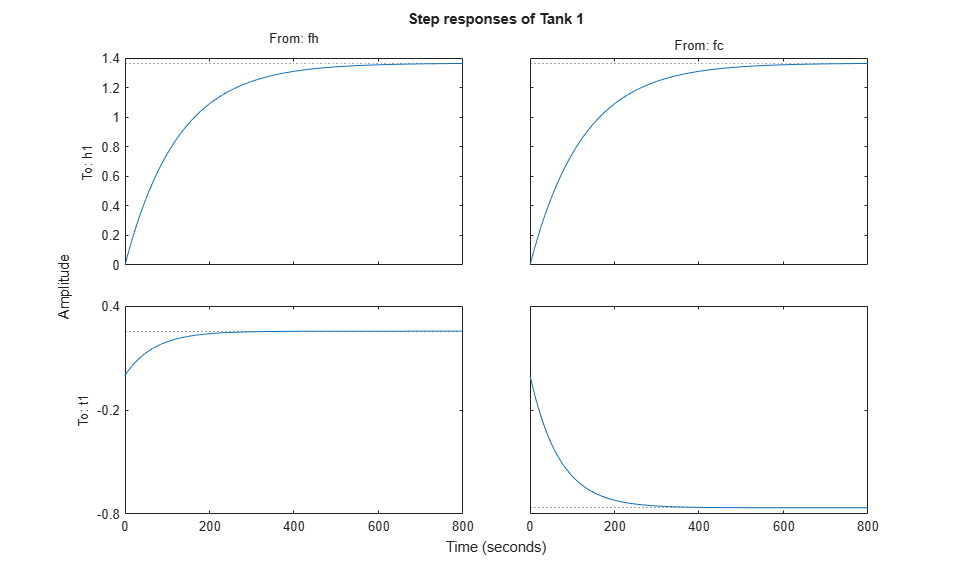Figure 2: Step responses of Tank 1.

The nominal model for tank 2 has inputs [|h1|;|t1|] and output `t2`:

```A = -(h1ss + beta + alpha*fb)/(A2*h2*alpha); B = [ (t2ss+t1ss)/(alpha*A2*h2), (h1ss + beta)/(alpha*A2*h2) ]; C = 1; D = zeros(1,2); tank2nom = ss(A,B,C,D,'InputName',{'h1','t1'},'OutputName','t2'); step(tank2nom), title('Step responses of Tank 2') ```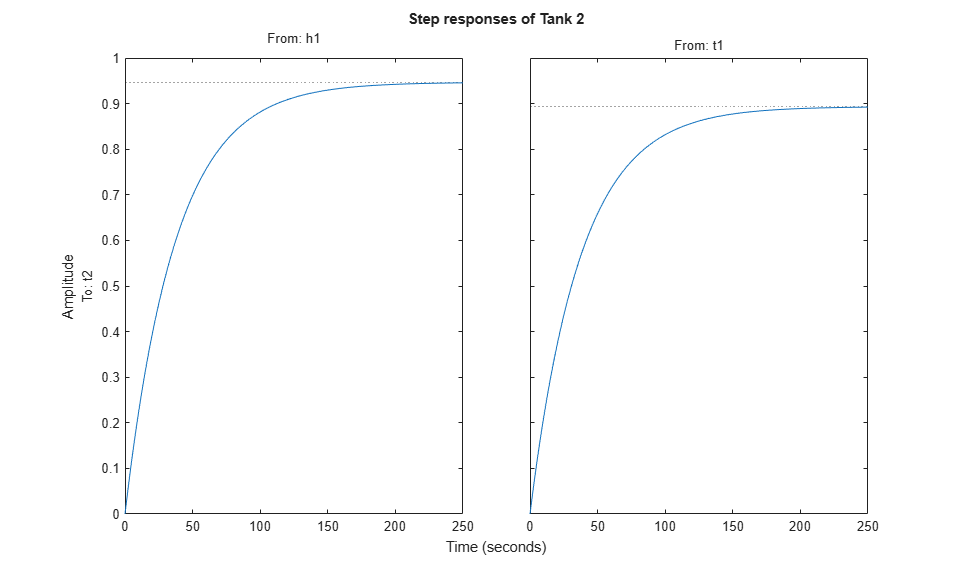Figure 3: Step responses of Tank 2.

### Actuator Models

There are significant dynamics and saturations associated with the actuators, so we'll want to include actuator models. In the frequency range we're using, we can model the actuators as a first order system with rate and magnitude saturations. It is the rate limit, rather than the pole location, that limits the actuator performance for most signals. For a linear model, some of the effects of rate limiting can be included in a perturbation model.

We initially set up the actuator model with one input (the command signal) and two outputs (the actuated signal and its derivative). We'll use the derivative output in limiting the actuation rate when designing the control law.

```act_BW = 20; % Actuator bandwidth (rad/sec) actuator = [ tf(act_BW,[1 act_BW]); tf([act_BW 0],[1 act_BW]) ]; actuator.OutputName = {'Flow','Flow rate'}; bodemag(actuator) title('Valve actuator dynamics') hot_act = actuator; set(hot_act,'InputName','fhc','OutputName',{'fh','fh_rate'}); cold_act =actuator; set(cold_act,'InputName','fcc','OutputName',{'fc','fc_rate'}); ```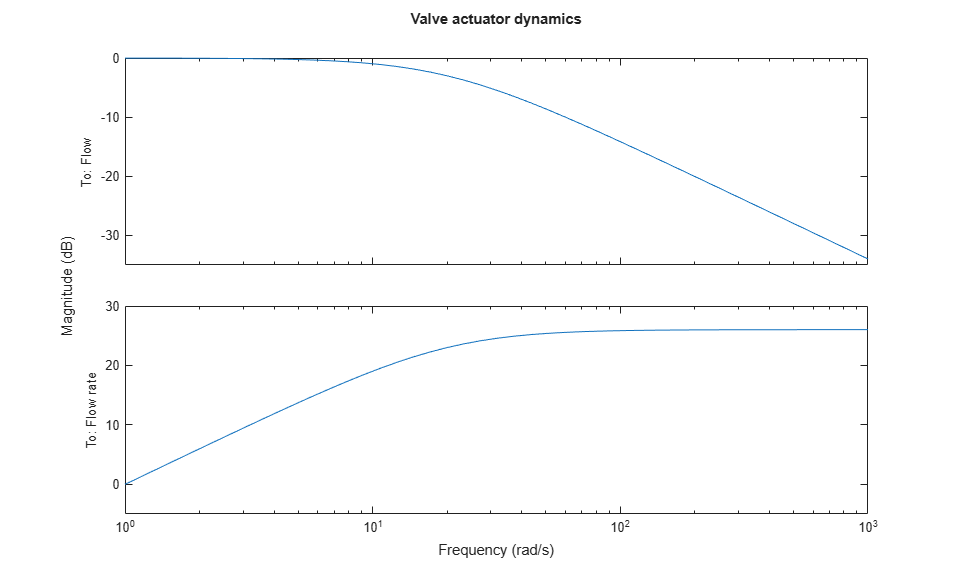Figure 4: Valve actuator dynamics.

### Anti-Aliasing Filters

All measured signals are filtered with fourth-order Butterworth filters, each with a cutoff frequency of 2.25 Hz.

```fbw = 2.25; % Anti-aliasing filter cut-off (Hz) filter = mkfilter(fbw,4,'Butterw'); h1F = filter; t1F = filter; t2F = filter; ```

### Uncertainty on Model Dynamics

Open-loop experiments reveal some variability in the system responses and suggest that the linear models are good at low frequency. If we fail to take this information into account during the design, our controller might perform poorly on the real system. For this reason, we will build an uncertainty model that matches our estimate of uncertainty in the physical system as closely as possible. Because the amount of model uncertainty or variability typically depends on frequency, our uncertainty model involves frequency-dependent weighting functions to normalize modeling errors across frequency.

For example, open-loop experiments indicate a significant amount of dynamic uncertainty in the `t1` response. This is due primarily to mixing and heat loss. We can model it as a multiplicative (relative) model error Delta2 at the `t1` output. Similarly, we can add multiplicative model errors Delta1 and Delta3 to the `h1` and `t2` outputs as shown in Figure 5.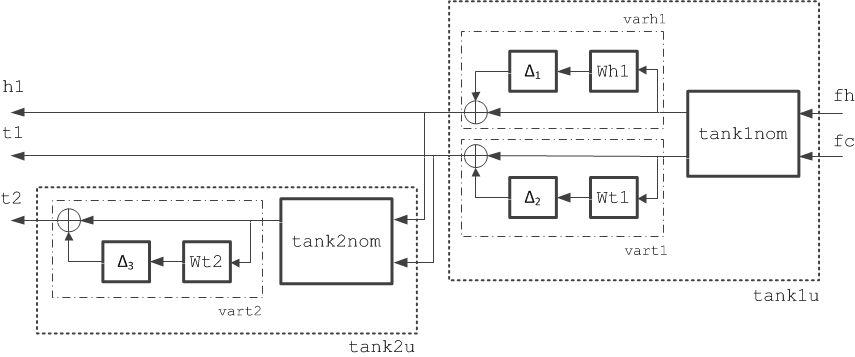Figure 5: Schematic representation of a perturbed, linear two-tank system.

To complete the uncertainty model, we quantify how big the modeling errors are as a function of frequency. While it's difficult to determine precisely the amount of uncertainty in a system, we can look for rough bounds based on the frequency ranges where the linear model is accurate or poor, as in these cases:

• The nominal model for `h1` is very accurate up to at least 0.3 Hz.

• Limit-cycle experiments in the `t1` loop suggest that uncertainty should dominate above 0.02 Hz.

• There are about 180 degrees of additional phase lag in the `t1` model at about 0.02 Hz. There is also a significant gain loss at this frequency. These effects result from the unmodeled mixing dynamics.

• Limit cycle experiments in the `t2` loop suggest that uncertainty should dominate above 0.03 Hz.

This data suggests the following choices for the frequency-dependent modeling error bounds.

```Wh1 = 0.01+tf([0.5,0],[0.25,1]); Wt1 = 0.1+tf([20*h1ss,0],[0.2,1]); Wt2 = 0.1+tf([100,0],[1,21]); clf bodemag(Wh1,Wt1,Wt2), title('Relative bounds on modeling errors') legend('h1 dynamics','t1 dynamics','t2 dynamics','Location','NorthWest') ```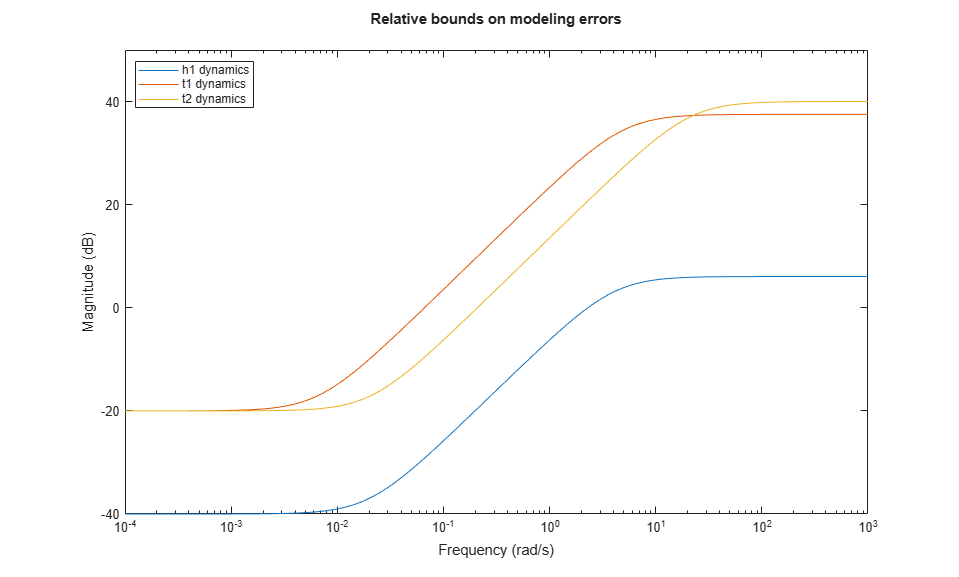Figure 6: Relative bounds on modeling errors.

Now, we're ready to build uncertain tank models that capture the modeling errors discussed above.

```% Normalized error dynamics delta1 = ultidyn('delta1',[1 1]); delta2 = ultidyn('delta2',[1 1]); delta3 = ultidyn('delta3',[1 1]); % Frequency-dependent variability in h1, t1, t2 dynamics varh1 = 1+delta1*Wh1; vart1 = 1+delta2*Wt1; vart2 = 1+delta3*Wt2; % Add variability to nominal models tank1u = append(varh1,vart1)*tank1nom; tank2u = vart2*tank2nom; tank1and2u = [0 1; tank2u]*tank1u; ```

Next, we randomly sample the uncertainty to see how the modeling errors might affect the tank responses

```step(tank1u,1000), title('Variability in responses due to modeling errors (Tank 1)') ```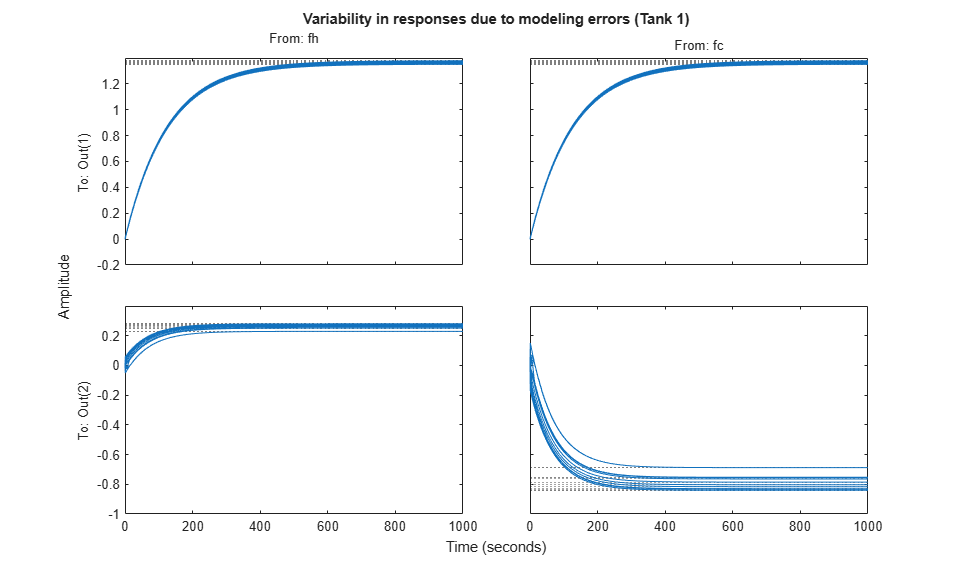Figure 7: Variability in responses due to modeling errors (Tank 1).

### Setting up a Controller Design

Now let's look at the control design problem. We're interested in tracking setpoint commands for `t1` and `t2`. To take advantage of H-infinity design algorithms, we must formulate the design as a closed-loop gain minimization problem. To do so, we select weighting functions that capture the disturbance characteristics and performance requirements to help normalize the corresponding frequency-dependent gain constraints.

Here is a suitable weighted open-loop transfer function for the two-tank problem: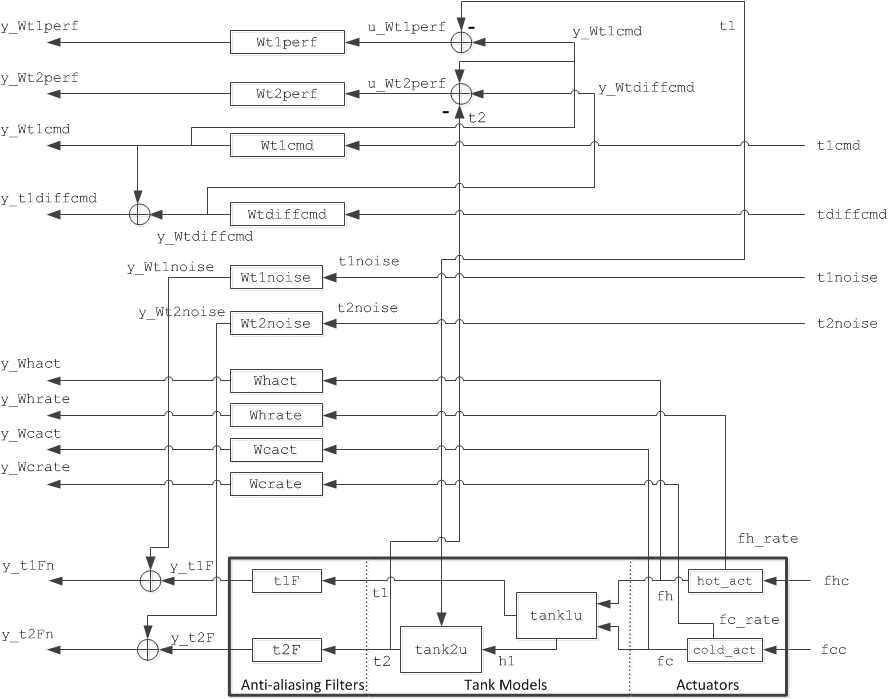Next, we select weights for the sensor noises, setpoint commands, tracking errors, and hot/cold actuators.

The sensor dynamics are insignificant relative to the dynamics of the rest of the system. This is not true of the sensor noise. Potential sources of noise include electronic noise in thermocouple compensators, amplifiers, and filters, radiated noise from the stirrers, and poor grounding. We use smoothed FFT analysis to estimate the noise level, which suggests the following weights:

```Wh1noise = zpk(0.01); % h1 noise weight Wt1noise = zpk(0.03); % t1 noise weight Wt2noise = zpk(0.03); % t2 noise weight ```

The error weights penalize setpoint tracking errors on `t1` and `t2`. We'll pick first-order low-pass filters for these weights. We use a higher weight (better tracking) for `t1` because physical considerations lead us to believe that `t1` is easier to control than `t2`.

```Wt1perf = tf(100,[400,1]); % t1 tracking error weight Wt2perf = tf(50,[800,1]); % t2 tracking error weight clf bodemag(Wt1perf,Wt2perf) title('Frequency-dependent penalty on setpoint tracking errors') legend('t1','t2') ```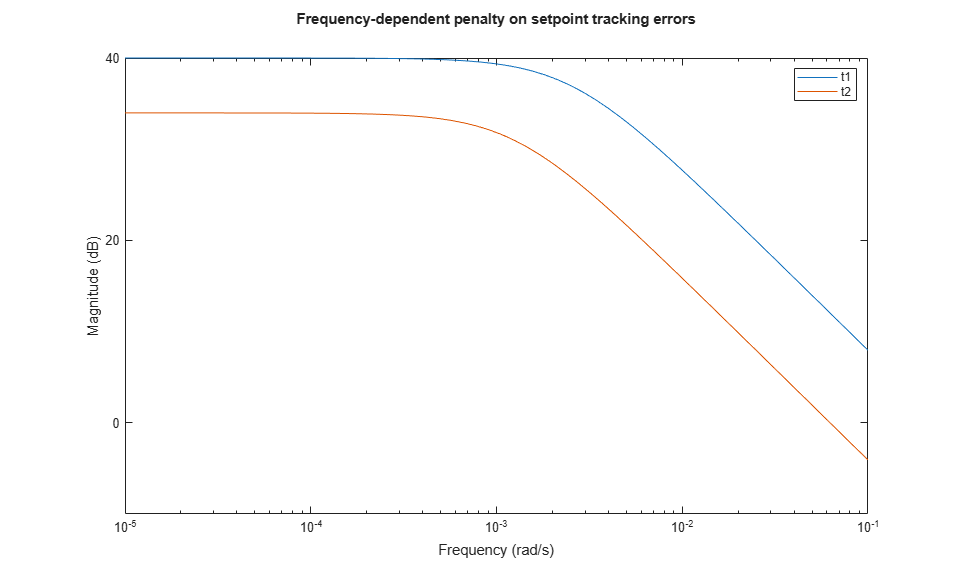Figure 9: Frequency-dependent penalty on setpoint tracking errors.

The reference (setpoint) weights reflect the frequency contents of such commands. Because the majority of the water flowing into tank 2 comes from tank 1, changes in `t2` are dominated by changes in `t1`. Also `t2` is normally commanded to a value close to `t1`. So it makes more sense to use setpoint weighting expressed in terms of `t1` and `t2-t1`:

` t1cmd = Wt1cmd * w1`
` t2cmd = Wt1cmd * w1 + Wtdiffcmd * w2`

where w1, w2 are white noise inputs. Adequate weight choices are:

```Wt1cmd = zpk(0.1); % t1 input command weight Wtdiffcmd = zpk(0.01); % t2 - t1 input command weight ```

Finally, we would like to penalize both the amplitude and the rate of the actuator. We do this by weighting `fhc` (and `fcc`) with a function that rolls up at high frequencies. Alternatively, we can create an actuator model with `fh` and d|fh|/dt as outputs, and weight each output separately with constant weights. This approach has the advantage of reducing the number of states in the weighted open-loop model.

```Whact = zpk(0.01); % Hot actuator penalty Wcact = zpk(0.01); % Cold actuator penalty Whrate = zpk(50); % Hot actuator rate penalty Wcrate = zpk(50); % Cold actuator rate penalty ```

### Building a Weighted Open-Loop Model

Now that we have modeled all plant components and selected our design weights, we'll use the `connect` function to build an uncertain model of the weighted open-loop model shown in Figure 8.

```inputs = {'t1cmd', 'tdiffcmd', 't1noise', 't2noise', 'fhc', 'fcc'}; outputs = {'y_Wt1perf', 'y_Wt2perf', 'y_Whact', 'y_Wcact', ... 'y_Whrate', 'y_Wcrate', 'y_Wt1cmd', 'y_t1diffcmd', ... 'y_t1Fn', 'y_t2Fn'}; hot_act.InputName = 'fhc'; hot_act.OutputName = {'fh' 'fh_rate'}; cold_act.InputName = 'fcc'; cold_act.OutputName = {'fc' 'fc_rate'}; tank1and2u.InputName = {'fh','fc'}; tank1and2u.OutputName = {'t1','t2'}; t1F.InputName = 't1'; t1F.OutputName = 'y_t1F'; t2F.InputName = 't2'; t2F.OutputName = 'y_t2F'; Wt1cmd.InputName = 't1cmd'; Wt1cmd.OutputName = 'y_Wt1cmd'; Wtdiffcmd.InputName = 'tdiffcmd'; Wtdiffcmd.OutputName = 'y_Wtdiffcmd'; Whact.InputName = 'fh'; Whact.OutputName = 'y_Whact'; Wcact.InputName = 'fc'; Wcact.OutputName = 'y_Wcact'; Whrate.InputName = 'fh_rate'; Whrate.OutputName = 'y_Whrate'; Wcrate.InputName = 'fc_rate'; Wcrate.OutputName = 'y_Wcrate'; Wt1perf.InputName = 'u_Wt1perf'; Wt1perf.OutputName = 'y_Wt1perf'; Wt2perf.InputName = 'u_Wt2perf'; Wt2perf.OutputName = 'y_Wt2perf'; Wt1noise.InputName = 't1noise'; Wt1noise.OutputName = 'y_Wt1noise'; Wt2noise.InputName = 't2noise'; Wt2noise.OutputName = 'y_Wt2noise'; sum1 = sumblk('y_t1diffcmd = y_Wt1cmd + y_Wtdiffcmd'); sum2 = sumblk('y_t1Fn = y_t1F + y_Wt1noise'); sum3 = sumblk('y_t2Fn = y_t2F + y_Wt2noise'); sum4 = sumblk('u_Wt1perf = y_Wt1cmd - t1'); sum5 = sumblk('u_Wt2perf = y_Wtdiffcmd + y_Wt1cmd - t2'); % This produces the uncertain state-space model P = connect(tank1and2u,hot_act,cold_act,t1F,t2F,Wt1cmd,Wtdiffcmd,Whact, ... Wcact,Whrate,Wcrate,Wt1perf,Wt2perf,Wt1noise,Wt2noise, ... sum1,sum2,sum3,sum4,sum5,inputs,outputs); disp('Weighted open-loop model: ') P ```
```Weighted open-loop model: Uncertain continuous-time state-space model with 10 outputs, 6 inputs, 18 states. The model uncertainty consists of the following blocks: delta1: Uncertain 1x1 LTI, peak gain = 1, 1 occurrences delta2: Uncertain 1x1 LTI, peak gain = 1, 1 occurrences delta3: Uncertain 1x1 LTI, peak gain = 1, 1 occurrences Type "P.NominalValue" to see the nominal value and "P.Uncertainty" to interact with the uncertain elements. ```

### H-infinity Controller Design

By constructing the weights and weighted open loop of Figure 8, we have recast the control problem as a closed-loop gain minimization. Now we can easily compute a gain-minimizing control law for the nominal tank models:

```nmeas = 4; % Number of measurements nctrls = 2; % Number of controls [k0,g0,gamma0] = hinfsyn(P.NominalValue,nmeas,nctrls); gamma0 ```
```gamma0 = 0.9016 ```

The smallest achievable closed-loop gain is about 0.9, which shows us that our frequency-domain tracking performance specifications are met by the controller `k0`. Simulating this design in the time domain is a reasonable way to check that we have correctly set the performance weights. First, we create a closed-loop model mapping the input signals [ `t1ref`; `t2ref`; `t1noise`; `t2noise`] to the output signals [ `h1`; `t1`; `t2`; `fhc`; `fcc`]:

```inputs = {'t1ref', 't2ref', 't1noise', 't2noise', 'fhc', 'fcc'}; outputs = {'y_tank1', 'y_tank2', 'fhc', 'fcc', 'y_t1ref', 'y_t2ref', ... 'y_t1Fn', 'y_t2Fn'}; hot_act(1).InputName = 'fhc'; hot_act(1).OutputName = 'y_hot_act'; cold_act(1).InputName = 'fcc'; cold_act(1).OutputName = 'y_cold_act'; tank1nom.InputName = [hot_act(1).OutputName cold_act(1).OutputName]; tank1nom.OutputName = 'y_tank1'; tank2nom.InputName = tank1nom.OutputName; tank2nom.OutputName = 'y_tank2'; t1F.InputName = tank1nom.OutputName(2); t1F.OutputName = 'y_t1F'; t2F.InputName = tank2nom.OutputName; t2F.OutputName = 'y_t2F'; I_tref = zpk(eye(2)); I_tref.InputName = {'t1ref', 't2ref'}; I_tref.OutputName = {'y_t1ref', 'y_t2ref'}; sum1 = sumblk('y_t1Fn = y_t1F + t1noise'); sum2 = sumblk('y_t2Fn = y_t2F + t2noise'); simlft = connect(tank1nom,tank2nom,hot_act(1),cold_act(1),t1F,t2F,I_tref,sum1,sum2,inputs,outputs); % Close the loop with the H-infinity controller |k0| sim_k0 = lft(simlft,k0); sim_k0.InputName = {'t1ref'; 't2ref'; 't1noise'; 't2noise'}; sim_k0.OutputName = {'h1'; 't1'; 't2'; 'fhc'; 'fcc'}; ```

Now we simulate the closed-loop response when ramping down the setpoints for `t1` and `t2` between 80 seconds and 100 seconds:

```time=0:800; t1ref = (time>=80 & time<100).*(time-80)*-0.18/20 + ... (time>=100)*-0.18; t2ref = (time>=80 & time<100).*(time-80)*-0.2/20 + ... (time>=100)*-0.2; t1noise = Wt1noise.k * randn(size(time)); t2noise = Wt2noise.k * randn(size(time)); y = lsim(sim_k0,[t1ref ; t2ref ; t1noise ; t2noise],time); ```

Next, we add the simulated outputs to their steady state values and plot the responses:

```h1 = h1ss+y(:,1); t1 = t1ss+y(:,2); t2 = t2ss+y(:,3); fhc = fhss/fs+y(:,4); % Note scaling to actuator fcc = fcss/fs+y(:,5); % Limits (0<= fhc <= 1) etc. ```

In this code, we plot the outputs, `t1` and `t2`, as well as the height `h1` of tank 1:

```plot(time,h1,'--',time,t1,'-',time,t2,'-.'); xlabel('Time (sec)') ylabel('Measurements') title('Step Response of H-infinity Controller k0') legend('h1','t1','t2'); grid ```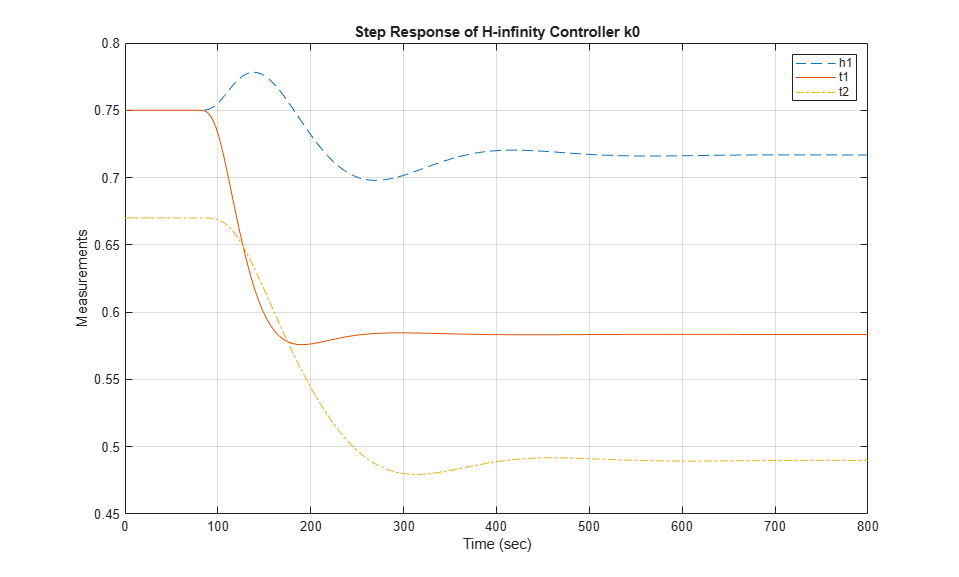Figure 10: Step response of H-infinity controller k0.

Next we plot the commands to the hot and cold actuators.

```plot(time,fhc,'-',time,fcc,'-.'); xlabel('Time: seconds') ylabel('Actuators') title('Actuator Commands for H-infinity Controller k0') legend('fhc','fcc'); grid ```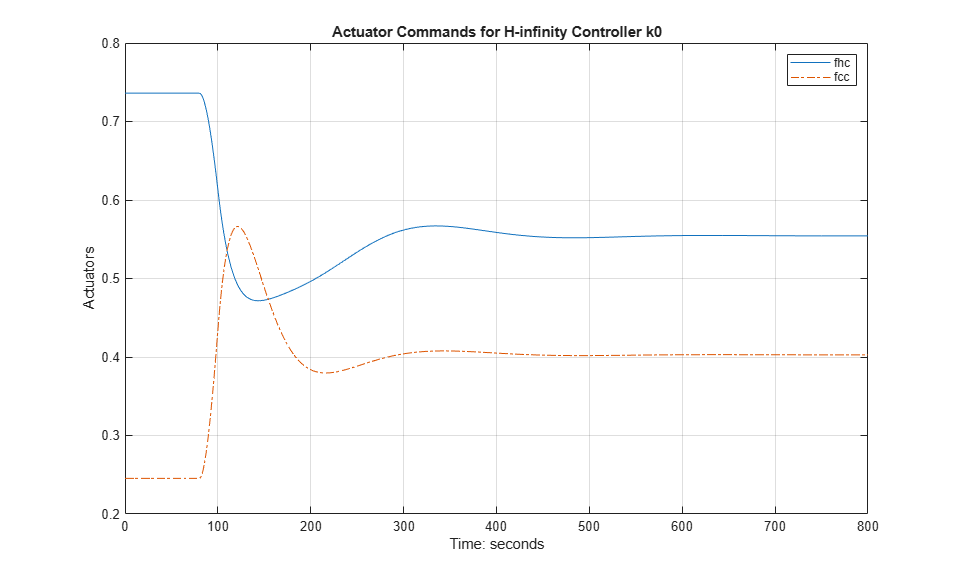Figure 11: Actuator commands for H-infinity controller k0.

### Robustness of the H-infinity Controller

The H-infinity controller `k0` is designed for the nominal tank models. Let's look at how well it fares for perturbed model within the model uncertainty bounds. We can compare the nominal closed-loop performance `gamma0` with the worst-case performance over the model uncertainty set. (see "Uncertainty on Model Dynamics" for more information.)

```clpk0 = lft(P,k0); % Compute and plot worst-case gain wcsigmaplot(clpk0,{1e-4,1e2}) ylim([-20 10]) ```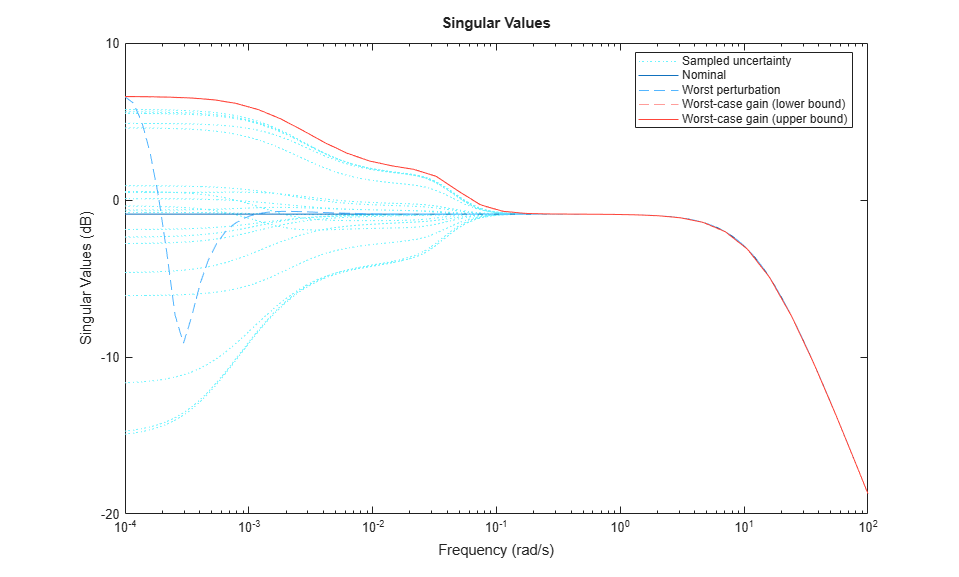Figure 12: Performance analysis for controller k0.

The worst-case performance of the closed-loop is significantly worse than the nominal performance which tells us that the H-infinity controller `k0` is not robust to modeling errors.

### Mu Controller Synthesis

To remedy this lack of robustness, we will use `musyn` to design a controller that takes into account modeling uncertainty and delivers consistent performance for the nominal and perturbed models.

```[kmu,bnd] = musyn(P,nmeas,nctrls); ```
``` D-K ITERATION SUMMARY: ----------------------------------------------------------------- Robust performance Fit order ----------------------------------------------------------------- Iter K Step Peak MU D Fit D 1 8.814 2.862 2.888 4 2 2.497 2.091 2.112 10 3 1.351 1.329 1.337 10 4 1.201 1.198 1.209 18 5 1.194 1.191 1.199 18 6 1.193 1.19 1.195 20 Best achieved robust performance: 1.19 ```

As before, we can simulate the closed-loop responses with the controller `kmu`

```sim_kmu = lft(simlft,kmu); y = lsim(sim_kmu,[t1ref;t2ref;t1noise;t2noise],time); h1 = h1ss+y(:,1); t1 = t1ss+y(:,2); t2 = t2ss+y(:,3); fhc = fhss/fs+y(:,4); % Note scaling to actuator fcc = fcss/fs+y(:,5); % Limits (0<= fhc <= 1) etc. % Plot |t1| and |t2| as well as the height |h1| of tank 1 plot(time,h1,'--',time,t1,'-',time,t2,'-.'); xlabel('Time: seconds') ylabel('Measurements') title('Step Response of mu Controller kmu') legend('h1','t1','t2'); grid ```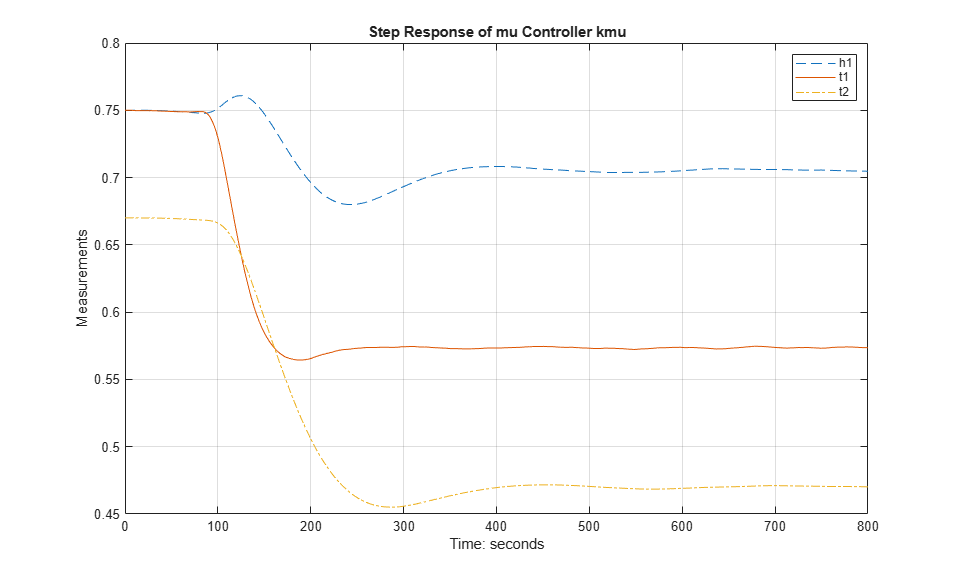Figure 13: Step response of mu controller kmu.

These time responses are comparable with those for `k0`, and show only a slight performance degradation. However, `kmu` fares better regarding robustness to unmodeled dynamics.

```% Worst-case performance for kmu clpmu = lft(P,kmu); wcsigmaplot(clpmu,{1e-4,1e2}) ylim([-20 10]) ```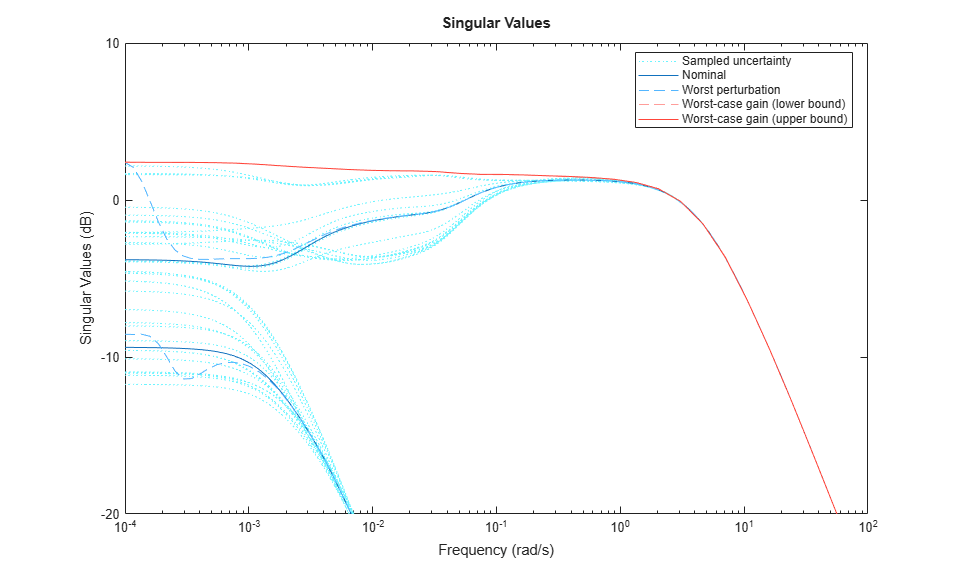Figure 14: Performance analysis for controller kmu.

You can use `wcgain` to directly compute the worst-case gain across frequency (worst-case peak gain or worst-case H-infinity norm). You can also compute its sensitivity to each uncertain element. Results show that the worst-case peak gain is most sensitive to changes in the range of `delta2`.

```opt = wcOptions('Sensitivity','on'); [wcg,wcu,wcinfo] = wcgain(clpmu,opt); wcg ```
```wcg = struct with fields: LowerBound: 1.3175 UpperBound: 1.3203 CriticalFrequency: 1.9273e-11 ```
```wcinfo.Sensitivity ```
```ans = struct with fields: delta1: 0 delta2: 60 delta3: 10 ```